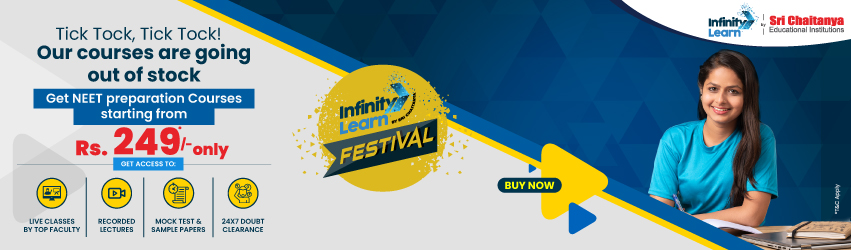NCERT Solutions for Class 11 Maths Chapter 8 Binomial Theorem Exercise 8.2

# NCERT Solutions for Class 11 Maths Chapter 8 Binomial Theorem Exercise 8.2Infinity Learn NEET Festival
Infinity Learn IIT JEE Festival

All 8.2 questions are answered in NCERT Solutions for Class 11 Maths Chapter 8 8.2 Binomial Theorem. The principals studied in Maths chapter 11 are the foundation for NCERT Solutions for Class 11 8.2. This practice is crucial for both the CBSE Term II exam and competitive exams. To do well on your exam, download these NCERT Solutions and practice them offline.

Need FREE NCERT/CBSE/IIT-JEE/NEET Study Material?

SOLUTIONS

NCERT Solutions for Class 11 Maths Chapter 8 Binomial Theorem 8.2

Using the NCERT solutions for 8.2 Class 11 Maths is the best option for the CBSE students when they are preparing for their exams. This chapter includes many exercises. One of them is Exercise 8.2 whose solutions are available in pdf format on this page. You can also download these solutions online from our website or app. Infinity Learn subject matter experts have designed solutions from the exercise with the utmost care and by keeping in mind all the guidelines by CBSE. Class 11 Students which have prepared with all the exercises given in this chapter can easily score well in the exams. By using these solutions, students came to know about the pattern of questions that are asked in the exam from this chapter and also know about the marks weightage of the chapter. So they can prepare well.

Along with this exercise, there are many number of exercises included in this chapter that contain innumerable questions as well. All these solutions are designed by subject matter experts as mentioned earlier. Hence all of these are considered as superior quality and students must refer to these solutions. To score well in the exam all these solutions must be practiced well and all other questions must be solved which are given next to it. Do not delay any more. Download the NCERT solutions for Class 11 Maths Chapter 8 8.2 from Infinity Learn website now for better exam preparation. If you have the Infinity Learn app on your phone, you should download the same through the app as well. You can use these solutions online and offline as well

1. Are NCERT Solutions for Class 11 Maths Chapter 8 2 exercise important from the exam point of view?

Ans. Yes, it is important for the students not to skip any exercise of this chapter from the NCERT textbook as all these exercises present in the NCERT book of Class 11 Maths are crucial for exams as well as for good marks. So, students must frequently practice all the questions present in NCERT Solutions for miscellanies exercise to make higher grades. These solutions are very easy to understand and are designed in simple language.

2. Is class 11 Maths chapter 5 easy?

Ans. This chapter can become easy for students if they have the correct mindset. They need correct guidance to get into the best path. The best guide today is Infinity Learn. Students can improve their scores by referring to these NCERT solutions. Students must use these NCERT Solutions to solve this chapter. The simplistic nature of the solution will help them to do the solutions with ease.

3. Explain the concept of the Binomial Theorem covered in Chapter 8 of NCERT Solutions for Class 11 Maths.

Ans. The Binomial theorem states, for positive integer n, whenever you add any two numbers, say a and b, the result raised to the power of n can also be written as the sum of (n+10 terms). The coefficients involved are expressed as binomial coefficients. The NCERT solutions of this chapter are provided on the Infinity Learn website and the app. Practice makes the man perfect, if you want to score well in them and in the other topics related to them, practice is the necessity.

Join Infinity Learn Regular Class Programme!